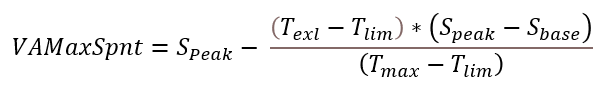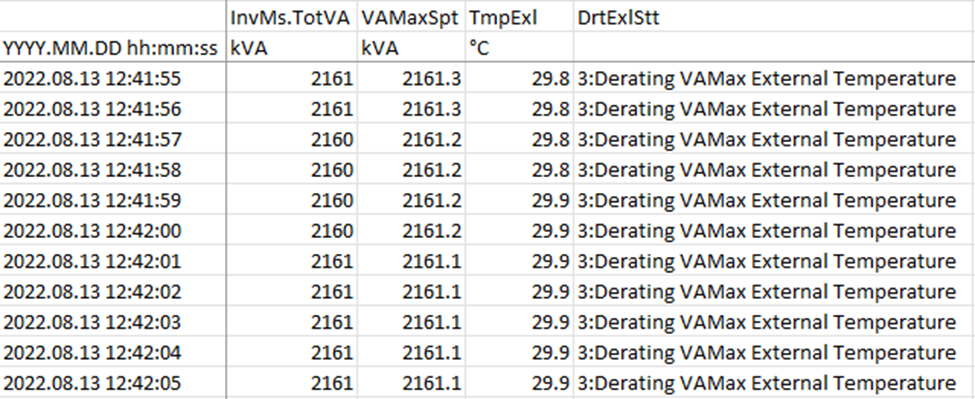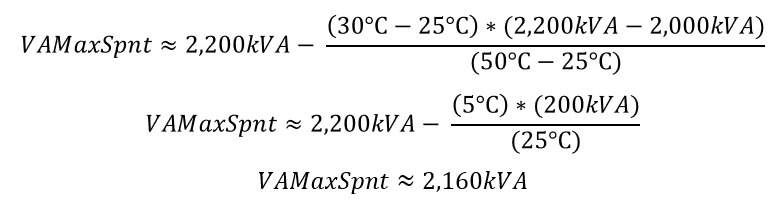# Kodiak External Temperature Derating / Loss of PowerThis article covers the external temperature derating characteristics of the SMA Kodiak series inverters.

The SMA Kodiak series inverters have a different function to achieve the nameplate kVA maximum when compared to older SMA models. The Kodiak series nameplate kVA value represents the inverter’s peak capabilities in optimum conditions, however the inverter commonly derates or curtails power levels. An inverter with the SC-2200-US nameplate, has a Base kVA Limit (Sbase) of 2,000kVA, but has a Peak kVA Limit or Nameplate kVA (Speak) at 2,200kVA during nominal conditions. The bonus power is standardized at 110% of the inverter’s Sbase.

Nominal conditions include many variables, but the most common to cause derating are voltage and temperature limits. When External Temperatures (Text) exceed the external Temperature Limits (Tlim) – which is typically standard at 25°C or 35°C by default – the inverter will begin to derate the bonus 10% kVA until the Maximum Value (Tmax)of 50°C is reached, and the inverter begins to critically derate.

This behavior can be observed and calculated using the inverter data. It is important to access the inverter’s log files directly. Other programs like the inverter Human Machine Interface (HMI) analysis page or SCADA may use aggregated data that does not accurately reflect the values. For example, the HMI may show maximum/minimum values over a 15-minute period, where the inverter log files show 1s instantaneous values. The inverter’s Derated Temperature kVA Maximum (VAMaxSpt – as listed in the inverter log files) can be approximated using the following function:(1)

When verifying a derate behavior, it is critical to check the DrtExlStt channel to see the derating status. Also, it is important to analyze a period where the inverter’s total apparent power (InvMs.TotVA) is reaching the derated limit (VAMaxSpt). For example, use the following excerpt from an SMA log file:Fig. 1 - Example SMA log file data set

We can deduce that the Tlim is 25°C, because the VAMaxSpt is derated below 2,200kVA and the temperature is above 25°C, but below 35°C. This behavior can also be observed using the plot function in the data.Fig. 2 - Example plot of SMA log file data from example set

In Fig. 2, observe that when the grey TmpExl line exceeds 25°C on the right-hand axis, the orange VAMaxSpt line reduces below 2,2200kVA on the left-hand axis. In the middle of the plot, an ideal data range can be observed where the blue InvMs.TotVA - inverter output - overlaps the orange VAMaxSpt. This is where the derating setpoint is active and prevents the inverter from exceeding the calculated VAMaxSpt limit.

Additionally, from the example data from Fig. 1, we can identify the following variables:

Texl ≈ 30°C (Found in the data channel TmpExl)

Tlim = 25°C (Deduced from inverter behavior)

Tmax = 50°C (This is standard)

Speak = 2,200kVA (At the top of the log file and nameplate value of inverter)

Sbase = 2,000kVA (Standard Speak divided by the decimal 110% bonus)

Using eq. (1)We genuinely hope this article was helpful. Now, help us stay sharp! Please share any notes you have so that we can keep supplying you with the most up-to-date information. Thanks and stay safe!

Did you find it helpful? Yes No

Send feedback